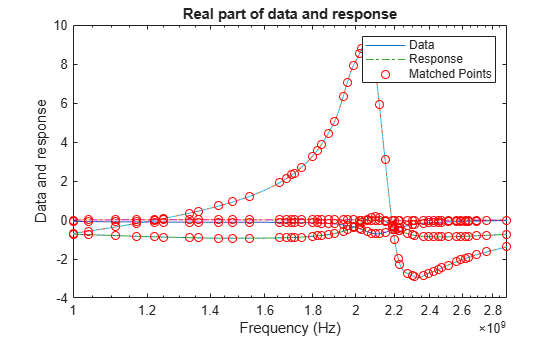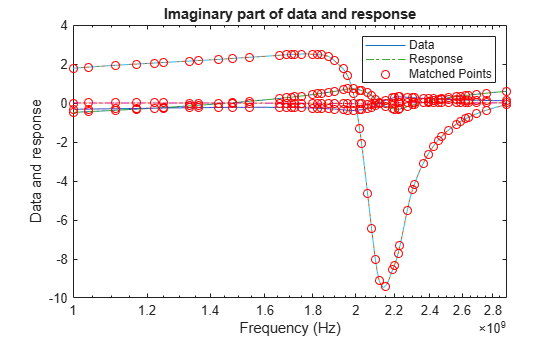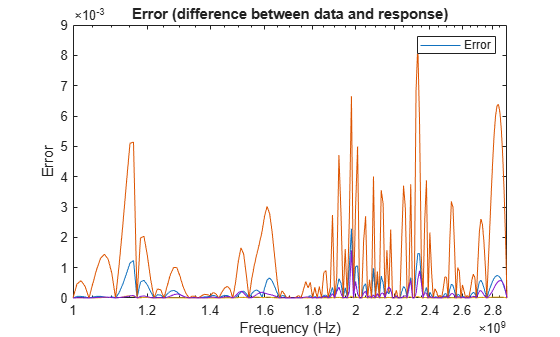# rational

Perform rational fitting to complex frequency-dependent data

## Description

Use the `rational` object and an interpolative algorithm to create a rational fit to frequency-dependant data.

The complex frequencies are given by the equation: $S=j2\pi freq$.

## Creation

### Syntax

``fit = rational(freq,data)``
``fit = rational(___,tol)``
``fit = rational(s,___)``
``[fit,error] = rational(___)``
``fit = rational(Name,Value)``

### Description

````fit = rational(freq,data)` returns a rational object with complex frequencies using the given frequency vector and network parameter data.```
````fit = rational(___,tol)` returns a rational object `fit` that satisfies a relative error tolerance.```

example

````fit = rational(s,___)` returns a rational object for N-port S-parameters.```
````[fit,error] = rational(___)` also returns the error of the fit.```
````fit = rational(Name,Value)` sets properties using one or more name-value pairs. For example, ```fit = rational('Tolerance',-34)```sets the relative error tolerance in decibels for the fit.```

### Input Arguments

expand all

Nonnegative frequencies, specified as a vector of nonnegative frequencies in Hz.

Data Types: `double`

Network parameter data, specified as a vector, a 2-D array or a 3-D array. The length of the data values must equal the length of the frequency values.

Relative error tolerance, specified as a scalar less than or equal to zero. `tol` value sets the input for the `'Tolerance'` property.

Data Types: `double`

N-port S-parameters, specified as an N-by-N matrix of elements of S sharing identical poles.

## Properties

expand all

Relative error tolerance, specified as a scalar less than or equal to zero.

Data Types: `double`

Behavior of fit for large S-parameters, specified as `true` or `false`. When `true`, the direct term in the fit is set to zero so that the rational fit `F(S)` tends to zero as S approaches infinity. When `false`, a nonzero direct term is allowed.

Data Types: `logical`

Maximum number of poles, specified as a scalar nonnegative integer.

Data Types: `double`

Display options for the fitting algorithm of the rational object, specified as one of the following:

• `'off'` - No display

• `'on'` - Printed information

• `'plot'` - Plots of the interpolation progress

• `'both'` - -Both printed information and plots.

Data Types: `char`

## Object Functions

 `zpk` Compute zeros, poles, and gain of rational object `ispassive` Return true if `rationalfit` output is passive at all frequencies `makepassive` Enforce passivity of `rationalfit` output or a rational object `freqresp` Frequency response of rational object and `rationalfit` function object `stepresp` Step-signal response of rational object and `rationalfit` function object `timeresp` Time response for rational object and `rationalfit` function object `passivity` Plot passivity of N-by-N `rationalfit` function output `generateSPICE` Generate SPICE file from `rationalfit` of S-parameters

## Examples

collapse all

Create s-paratmeters from `passive.s2p`.

`S = sparameters('passive.s2p');`

Perform rational fitting of the S-parameters.

`fit = rational(S);`

Create a S-Parameters object from the file named `default.s2p`. Perform rational fitting of the S-Parameters.

```S = sparameters('default.s2p'); fit = rational(S,'Display', 'plot')``````fit = rational with properties: NumPorts: 2 NumPoles: 25 Poles: [25x1 double] Residues: [2x2x25 double] DirectTerm: [2x2 double] ErrDB: -21.7113 ```

Calculate the zeros, poles, gain, and DC gain of the rational object.

`[z,p,k,dcgain] = zpk(fit)`
```z=2×2 cell array {25x1 double} {25x1 double} {25x1 double} {25x1 double} ```
```p=2×2 cell array {25x1 double} {25x1 double} {25x1 double} {25x1 double} ```
```k = 2×2 0.8240 0.0039 -0.0405 0.0011 ```
```dcgain = 2×2 0.9248 -0.0108 -1.2311 0.8725 ```